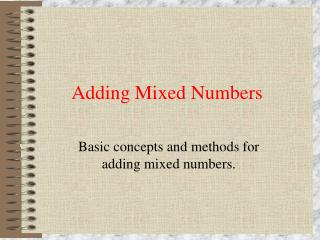DownloadDownload PresentationDownload Presentation- - - - - - - - - - - - - - - - - - - - - - - - - - - E N D - - - - - - - - - - - - - - - - - - - - - - - - - - -
##### Presentation Transcript

1. Adding Mixed Numbers Basic concepts and methods for adding mixed numbers.

2. Definition • Mixed Number = A mixed number is a whole number with a fraction attached. • Example = 3 4/5, This is read as three and four fifths.

3. Addition of mixed numbers with like denominators. If James has two and one eighth pizzas and Jane has two and five eighths pizzas, how many pizza’s do they have together. 2 1/8 + 2 5/8 Step 1: Add the Fractions 1/8 + 5/8 6/8

4. Addition of mixed numbers with like denominators. If James has two and one eighth pizzas and Jane has two and five eighths pizzas, how many pizza’s do they have together. Step 2: Add the whole numbers 2 + 2 4

5. Addition of mixed numbers with unlike denominators. If James has two and one eighth pizzas and Jane has two and three fourths pizzas, how many pizza’s do they have together. 2 1/8 + 2 3/4 1/8 + 6/8 Step 1:Find a common denominator. For this problem we will use 8, since 8 is a multiple of 4. 1/8 + 6/8 Step 2: Add the Fractions 7/8

6. Addition of mixed numbers with unlike denominators. If James has two and one eighth pizzas and Jane has two and three fourths pizzas, how many pizza’s do they have together. Step 3: Add the whole numbers 2 + 2 4

7. Addition of mixed numbers with unlike denominators. Step 4: Combine the whole number and the fraction. 2 + 7/8 2 7/8

8. Adding Mixed Numbers Presented By: Michael Lee Ratcliff II